International
Tables for
Crystallography
Volume A
Space-group symmetry
Edited by M. I. Aroyo

International Tables for Crystallography (2016). Vol. A, ch. 3.2, pp. 738-739

Section 3.2.2.1.3. Enantiomorphism, enantiomerism, chirality, dissymmetry

H. Klappera and Th. Hahna

3.2.2.1.3. Enantiomorphism, enantiomerism, chirality, dissymmetry

| top | pdf |

All these terms refer to the same symmetry restriction, the absence of improper rotations (rotoinversions, rotoreflections) in a crystal or in a molecule. This implies in particular the absence of a centre of symmetry,, and of a mirror plane,, but also of aaxis. As a consequence, such chiral crystals or molecules can occur in two different forms, which are related as a right and a left hand; hence, they are called right-handed and left-handed forms. These two forms of a molecule or a crystal are mirror-related and not superimposable (not congruent). Thus, the only symmetry operations that are allowed for chiral objects are proper rotations. Such objects are also called dissymmetric, in contrast to asymmetric objects, which have no symmetry.

The terms enantiomerism and chirality are mainly used in chemistry and applied to molecules, whereas the term enantiomorphism is preferred in crystallography if reference is made to crystals.

Enantiomorphic crystals, as well as solutions or melts containing chiral molecules of one handedness, exhibit optical activity (cf. Section 3.2.2.4.2). Crystals and molecules of the other handedness show optical activity with the opposite sense of rotation. For this reason, two molecules of opposite chirality are also called optical isomers.

Chiral molecules form enantiomorphic crystals of the corresponding handedness. These crystals belong, therefore, to one of the 11 crystal classes allowing enantiomorphism (Table 3.2.2.1). Racemic mixtures (containing equal amounts of molecules of each chirality), however, may crystallize in non-enantiomorphic or even centrosymmetric crystal classes. Racemization (i.e. the switching of molecules from one chirality to the other) of an optically active melt or solution can occur in some cases during crystallization, leading to non-enantiomorphic crystals.

 Table 3.2.2.1| top | pdf | The 11 Laue classes, the 21 noncentrosymmetric crystallographic point groups (crystal classes) and the occurrence (+) of specific crystal properties
 The number of non-zero independent tensor components is given in parentheses. For comparison, the icosahedral and the sphere groups (isotropy) are added. For first-rank tensors the direction [uvw] of the property vector is given. There are 10 pyroelectric, 20 piezoelectric, 11 enantiomorphic and 15 optically active crystal classes.
Crystal systemLaue classNoncentrosymmetric point groupFirst-rank tensor (e.g. pyroelectricity, piezoelectricity under hydrostatic pressure)Third-rank tensor (e.g. piezoelectricity, second-harmonic generation)EnantiomorphismAxial second-rank tensor (optical activity = optical gyration)
Triclinic1 + (3) [uvw] + (18) + + (6)
Monoclinic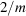2 + (1)  + (8) + + (4)
m + (2) [u0w] + (10) + (2)
Orthorhombic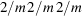222 + (3) + + (3)
mm2 + (1)  + (5) + (1)
Tetragonal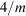4 + (1)  + (4) + + (2)+ (4) + (2)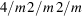422 + (1) + + (2)
4mm + (1)  + (3)+ (2) + (1)
Trigonal3 + (1)  + (6) + + (2)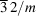32 + (2) + + (2)
3m + (1)  + (4)
Hexagonal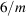6 + (1)  + (4) + + (2)+ (2)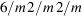622 + (1) + + (2)
6mm + (1)  + (3)+ (1)
Cubic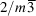23 + (1) + + (1)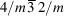432 + + (1)+ (1)

Icosahedral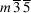(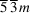) 235 (532) + + (1)
Spherical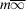(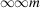)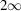(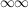) + + (1)
Unique axis b.
Hexagonal axes.

Enantiomorphic crystals can also be built up from achiral molecules or atom groups. In these cases, the achiral molecules or atom groups form chiral configurations in the structure. The best known examples are quartz and NaClO3. For details, reference should be made to Rogers (1975).

References

Rogers, D. (1975). Some fundamental problems of relating tensorial properties to the chirality or polarity of crystals. In Anomalous Scattering, edited by S. Ramaseshan & S. C. Abrahams, pp. 231–250. Copenhagen: Munksgaard.